# Community

Blog Events Webinars Tutorials Forum
×
Community Blog Machine Learning based Web Exception Detection

# Machine Learning based Web Exception Detection

The next-generation web intrusion detection technology based on machine learning technology is expected to make up for the deficiency in the traditional rule set approach.Web firewalls are the first line of defense when it comes to information security. With rapid updates in network technologies, new hacking tricks are also emerging, bringing forth challenges for firewalls following traditional rules. Traditional web intrusion detection techniques intercept intrusion access by maintaining rule sets. On the one hand, hard rules are easy to bypass by sly hackers, and rule sets based on common knowledge have difficulty coping with 0-day attacks. On the other hand, there is a high threshold and there are high costs for defenders to set up and maintain rules.

The next-generation web intrusion detection technology based on machine learning technology is expected to make up for the deficiency in the traditional rule set approach, and bring new developments and breakthroughs for defenders in the web confrontation.

Machine learning methods can carry out automated learning and training based on massive data and have seen wide application to image, voice, and natural language processing as well as other aspects.

However, there are challenges to apply machine learning to web intrusion detection, the biggest one being the lack of label data. Despite the large amount of normal access traffic data, web intrusion samples are scarce and varied, making it difficult to learn and train models. Therefore, most of the current web intrusion detection approaches rely on unsupervised methods, naming establishing profiles for a large number of normal logs, and accesses that are at variance with normal traffic - identified as exceptions. The idea behind this is just the opposite to the construction of interception rules. Interception rules aim to identify intrusions, and thus need to "trim the sails" in confrontation. However, the profile-based approach is, by design, intended to profile normal traffic, to "meet changes with constancy" in confrontation, and thus is more difficult to bypass.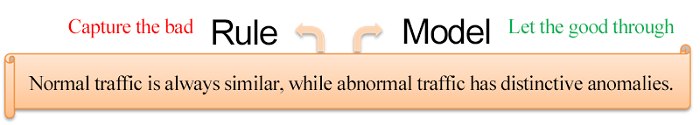Exception detection-based web intrusion recognition requires to abstract the URL-specific statistics or machine learning profiles that can describe the sample sets based on a large number of normal samples in the training phase. In the detection phase, the approach identifies the exception by determining whether the web access is in line with the profile.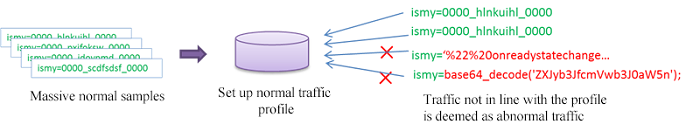## There are several key ideas for establishing a profile, mentioned below:

1. Statistics-based learning model
Statistics-based learning web exception detection usually requires numerical extraction and an analysis of the features of normal data traffic. Features include the number of URL parameters, the mean and variance of the length of the parameter value, the parameter character distribution, the access frequency of the URL, and so on. Furthermore, it requires the establishment of mathematical models through statistical analysis on the distribution of features in a large number of samples, and exception detection to proceed via statistical methods.

2. Text analysis-based machine learning model
Web exception detection is a part of the final analysis based on the analysis of log text, thus we can learn some of the ideas in NLP for text analysis and modeling. Specifically, one of the successful practices is the Hidden Markov Model (HMM)-based parameter value exception detection.

3. The one-class model-based
As black samples of web intrusions are few, it is hard to conduct training in traditional supervised learning methods. The white sample-based exception detection, however, enables sample learning through unsupervised or one-class models to construct the minimal model that can completely express the white samples as the profile for exception detection.

4. The clustering model-based
In general, normal traffic has quite a repetition of visitors, with extremely rare intrusion actions. Therefore, through clustering analysis on web access, we can catch hold of a small number of exceptions amongst a large number of normal behaviors to identify intrusions.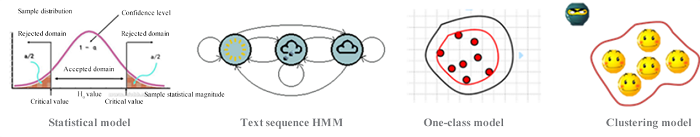## Statistics-based learning model

The statistics-based learning model approach mainly involves establishing a feature set for the data, and then carrying out statistical modeling for each feature. For the testing sample, first calculate the abnormality degree of every feature, then use the model to fuse and grade the outliers, and finally treat the results as the final basis for exception detection.

Here we take an excerpt from the Stanford University CS259D: Data Mining for Cyber Security course  as an example to introduce some effective features and exception detection methods.

### Feature 1: Length of parameter value

Model: The length distribution
The mean value is μ, and the variance is σ2. Use Chebyshev's inequality to calculate the outlier p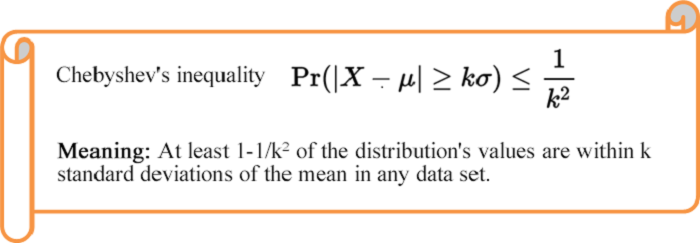### Feature 2: Character distribution

Model: Create a model for character distribution
Use a chi-squared test to calculate the outlier p.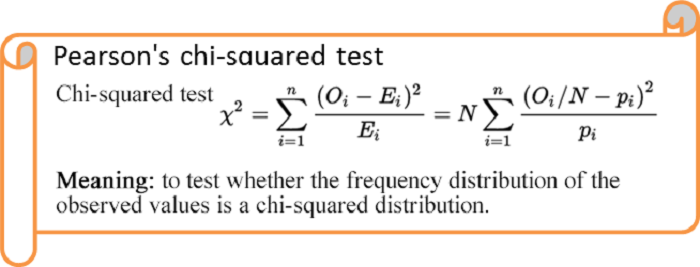### Feature 3: Missing parameter

Model: Create a parameter table
Check erroneous or missing parameters through the look-up table method.

### Feature 4: Parameter sequence

Model: Directed graph of the order of parameters to determine whether there is a violation of the order.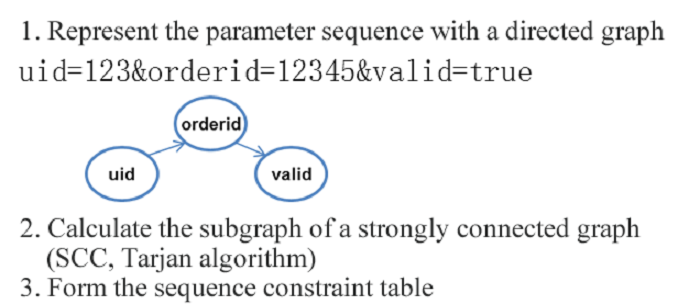### Feature 5: Access frequency (the access frequency of an individual IP address, and the total access frequency)

Model: The access frequency distribution within a given period.
The mean is μ, and the variance is σ2. Use Chebyshev's inequality to calculate the outlier p.

### Feature 6: Access interval

Model: The interval time distribution. Use a chi-squared test to calculate the outlier p.
Finally, use multiple feature outliers to fuse the exception grading model to get the final exception score: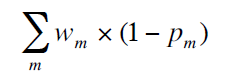## Text analysis-based machine learning model

Behind the URL parameter input is the background code parsing. In general, the value of each parameter has a range, and the allowable input follows a certain pattern. See the example below:In the example, the green logs represent the normal traffic flows, and the red represents the abnormal traffic flow. As the abnormal traffic flow has very similar parameters, value length, and character distributions to those of the normal traffic flows, using the above feature statistical method, it is difficult to tell them apart. In addition, the normal traffic flows differ from one another, but they share a common pattern, while the abnormal traffic does not match the pattern. In this example, the sample pattern that matches the value is: numbers_letters_numbers, and we can use a state machine to express the valid value range: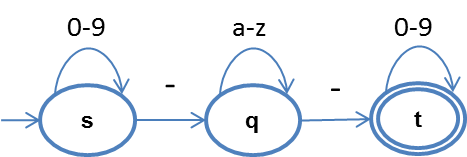The text sequence pattern modeling is more accurate and reliable than numerical features. Among these, a suitable application is the sequence modeling based on Hidden Markov Model (HMM), which we will briefly introduce here.

In the HMM-based state sequence modeling, first, you need to convert the original data to a state. For example, 'N' represents the number to indicate the state, and 'a' represents the letter to indicate the state, while other characters remain unchanged. This step can also be the normalization of the original data. The result is that we have effectively managed to compress the state space of the original data and have further reduced the gap between normal samples.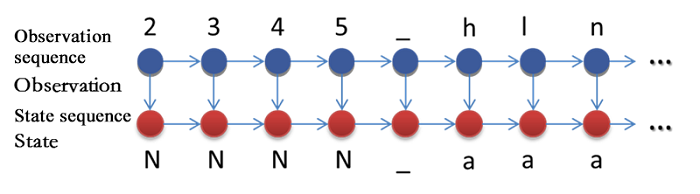As the next step, we calculate the probability distribution of the state after each state. For example, a possible result is as depicted in the figure below. The "^" represents the begin symbol. Since white samples all start with numbers, the probability of the start symbol (state ^) to shift to the number (state N) is 1. Now, the next state of the number (state N) has a probability of 0.8 to be a number (state N) again, a probability of 0.1 to shift to an underscore, and a probability of 0.1 to shift to the terminator (state \$), and so on.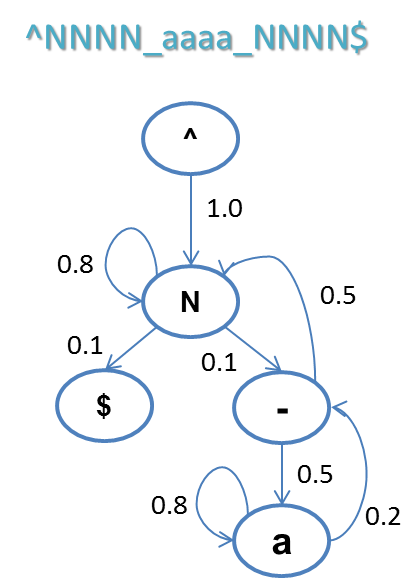Using this state transition model, we can determine whether an input sequence conforms to the white sample pattern: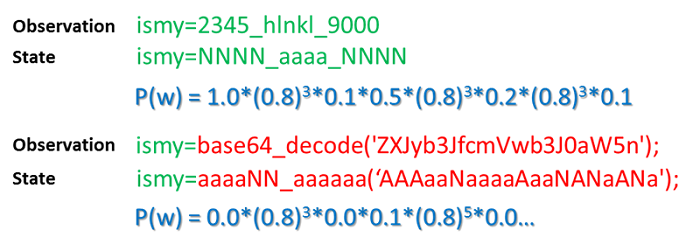The occurrence probability of the state sequence of normal samples is higher than that of the abnormal samples. You can perform the abnormity identification by setting an appropriate threshold.

## The one-class model-based

In binary classification issues, since we only have a large number of white samples, we can consider the one-class models to learn the minimum frontier of the single-class samples. Accesses outside of the frontier will fall in the exceptions category.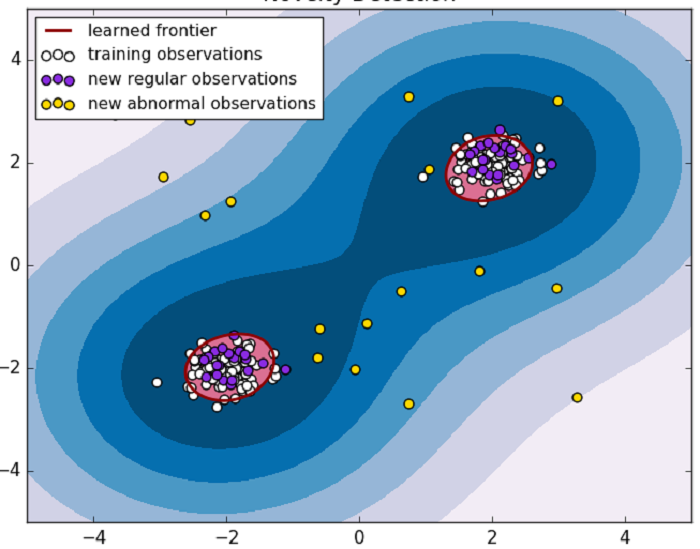A successful application among such methods is the one-class support vector machine (one-class SVM). Here is a brief introduction of the idea of McPAD, a successful case utilizing this method.

McPAD system first vectorizes the text data through the N-Gram, as in the example below:First, divide the text into N-Gram sequences by a sliding window of length N. In this example, N is 2 and the sliding window step length is 1. You will obtain the following N-Gram sequence.In the next step, we will convert the N-Gram sequence into a vector. Suppose there are 256 different characters, and we will get 256 * 256 kinds of 2-GRAM combinations (such as aa, ab, ac...). We can use a 256 * 256 long vector, and each bit is one-hot encoded (1 for a high bit and 0 for a low bit) to represent whether the 2-GRAM appears in the text. Then we will get a 256 * 256 long 0/1 vector. In the next step, we use the occurrence frequency of this 2-Gram in the text to replace the monotonous "1" for each 2-Gram to deliver more information: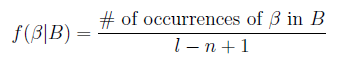At this point, we can represent each text by a 256 * 256 long vector.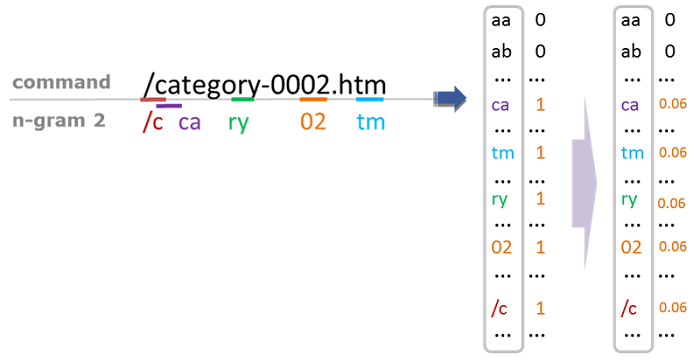Now we get the 256 * 256-vector set of the training sample, and need to identify the minimal frontier through the one-class SVM. The problem, however, is that the dimension size of the sample is too high, posing a difficulty in training. Another problem that needs a solution is reduction in the feature dimensionality. There are many mature methods in feature dimension reduction, and the McPAD system adopts the clustering approach for features to reduce the dimensionality.

## The clustering model-based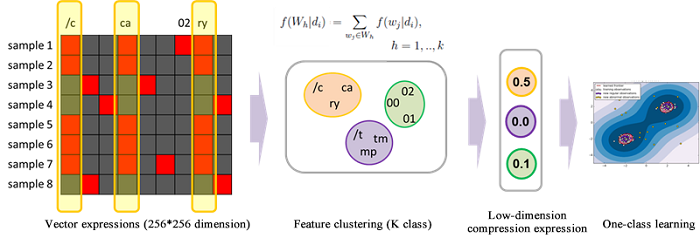In the upper left matrix, black blocks represent 0 and red blocks represent nonzero values. Each row in the matrix represents the 2-Grams in each input text (sample). If we look at this matrix from another angle, each column represents the samples existing in a 2-Gram. Therefore, we can express each 2-Gram by the sample vector. From this perspective, we can get 2-Gram relevance. In the 2-Gram vector clustering, the specified number of classes 'K' is the number of feature dimensions present after dimension reduction. We can then apply the reduced feature vectors into the one-class SVM for further model training.

Furthermore, you can replace the process of McPAD adopting the linear feature reduction + one-class SVM to address the white model training by the in-depth auto-encoding model in deep learning to conduct nonlinear feature reduction. At the same time, the auto-encoding model training process entails learning the compression expression of the training sample and determining whether the input sample is consistent with the model through the reconstruction error of the given input.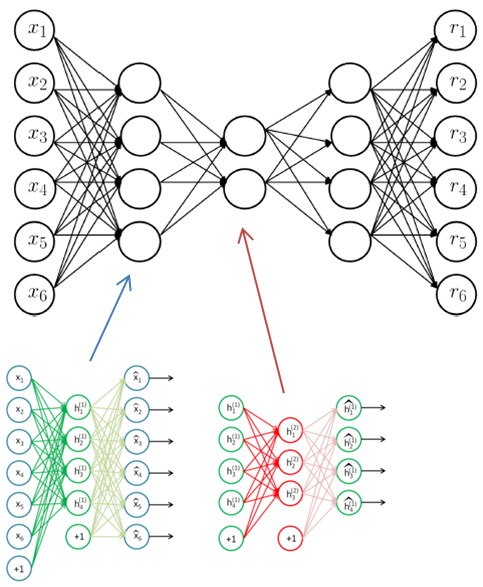Now, we will continue with the text vectorization method of McPAD through 2-Gram to directly input the vectors into the in-depth auto-encoding model for training. In the test phase, we will calculate the reconstruction error to serve as the criterion for exception detection.Based on this framework, the basic flow of exception detection is as follows.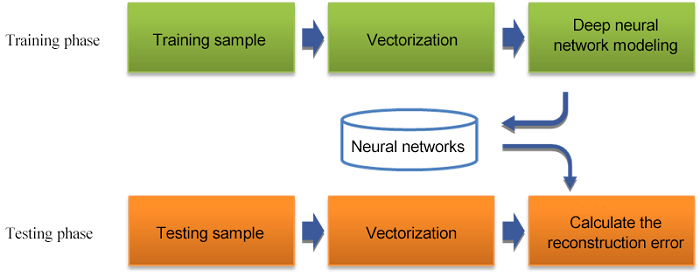## Summary

This article is a glimpse of several ideas for leveraging machine learning in web exception detection. Web traffic exception detection is only a part of the web intrusion detection, and serves to catch a small amount of "suspicious" behaviors from the massive logs. However, numerous false alerts also arise in this "small amount", and the approach is far from being capable of directly delivering in WAF direct interception. A sound web intrusion detection system should also incorporate intrusion identification on this basis, as well as false alerts reduction.

1 1 1
Share on

### You may also like## Raja_KT February 16, 2019 at 6:20 am

Good one. If you can tell more on the price of what we have to pay on routing volume of traffics....and how to address....

# Related Products

• ##Machine Learning Platform For AI

An end-to-end platform that provides various machine learning algorithms to meet your data mining and analysis requirements.

• ##Epidemic Prediction Solution

This technology can be used to predict the spread of COVID-19 and help decision makers evaluate the impact of various prevention and control measures on the development of the epidemic.

• ##Machine Translation

Relying on Alibaba's leading natural language processing and deep learning technology.

• ##Starter Package

High-performance virtual machines with data transfer plan, starting from \$2.50 per month